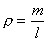EXAMPLE 3: MASS MOMENT OF INERTIA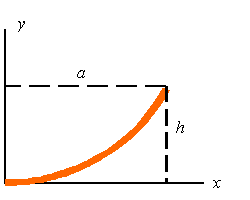Calculate the mass moment of inertia of the parabolic rod about the y-axis. Assume the rod is made of a uniform material and has a mass of m.

Solution:

The mass moment of inertia about the y-axis is given by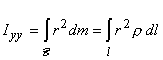The length of the bar can be calculated from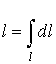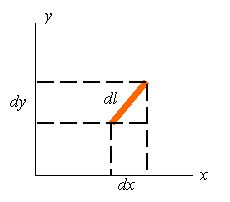The element of arc length in a rectangular coordinate system can be written as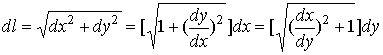The equation for the parabola is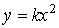Substitution of the point (a, h) into this equation givens the equation of the bar as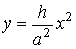The length of the bar can, therefore, be calculated as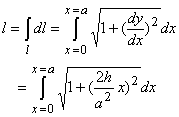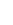The distance from the y-axis is x. Therefore, r=x. The mass moment of inertia about the y-axis can be written as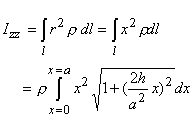For a uniform bar the density can be calculated using the total mass and total length of the bar so that